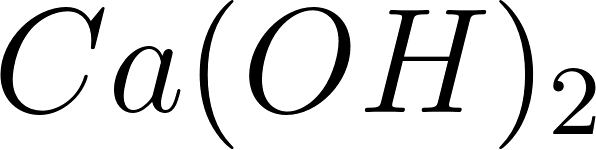×
Get Full Access to Introductory Chemistry - 5 Edition - Chapter 14 - Problem 30p
Get Full Access to Introductory Chemistry - 5 Edition - Chapter 14 - Problem 30p

×

# Answer: Identify each substance as an acid or a base and write a chemical equationISBN: 9780321910295 34

## Solution for problem 30P Chapter 14

Introductory Chemistry | 5th Edition

• Textbook Solutions
• 2901 Step-by-step solutions solved by professors and subject experts
• Get 24/7 help from StudySoup virtual teaching assistantsIntroductory Chemistry | 5th Edition

4 5 1 248 Reviews
31
3
Problem 30P

Problem 30P

Identify each substance as an acid or a base and write a chemical equation showing how it is an acid or a base according to the Arrhenius definition.

(a) Ca(OH)2(aq)

(b) HC2H3O2(aq)

(c) KOH(aq)

(d) HNO3(aq)

Step-by-Step Solution:

Problem 30P

Identify each substance as an acid or a base and write a chemical equation showing how it is an acid or a base according to the Arrhenius definition.

(a) Ca(OH)2(aq)

(b) HC2H3O2(aq)

(c) KOH(aq)

(d) HNO3(aq)

Step by step solution

Step 1 of 4

(a)

Arrhenius acid: The substance which produces hydronium ion in aqueous solution.

Arrhenius base: The substance which produces hydroxide ions in  an aqueous solution.

(a)

The givenTheionizes in water to produceion. The chemical reaction is as follows.Therefore, it is an Arrheneius base.

_________________________________________________________________________

Step 2 of 4

Step 3 of 4

##### ISBN: 9780321910295

This full solution covers the following key subjects: acid, base, equation, according, chemical. This expansive textbook survival guide covers 19 chapters, and 2046 solutions. The full step-by-step solution to problem: 30P from chapter: 14 was answered by , our top Chemistry solution expert on 05/06/17, 06:45PM. Since the solution to 30P from 14 chapter was answered, more than 497 students have viewed the full step-by-step answer. The answer to “Identify each substance as an acid or a base and write a chemical equation showing how it is an acid or a base according to the Arrhenius definition.(a) Ca(OH)2(aq)(b) HC2H3O2(aq)(c) KOH(aq)(d) HNO3(aq)” is broken down into a number of easy to follow steps, and 32 words. This textbook survival guide was created for the textbook: Introductory Chemistry, edition: 5. Introductory Chemistry was written by and is associated to the ISBN: 9780321910295.

Unlock Textbook Solution